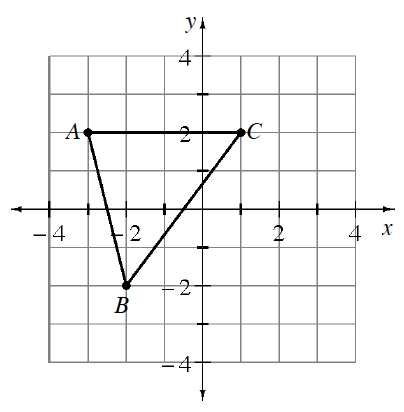Home > CCAA > Chapter cc36 > Lesson cc36.2.1 > Problem6-46

6-46.

Louis is dilating triangle ABC below. He multiplied each $x$-coordinate and $y$-coordinate of triangle ABC by $-2$. 6-46 HW eTool (Desmos). Homework Help ✎1. What are the new coordinates of the points?

Use the graph to find the coordinates of points A, B, and C. Then multiply each value by $−2$. For example, point A is originally $(−3,2)$. Its new coordinates will be $(6,−4)$. Find points B and C.

2. Graph Louis’ new triangle.

After multiplying each $x$-coordinate and $y$-coordinate by $−2$, plot the new points.

3. Describe how triangle ABC changed.

Its sides are twice as long and rotated $180°$.

Use the eTool below to graph Louis' new triangle.
Click the link at right for the full version of the eTool: CC3 6-46 HW eTool